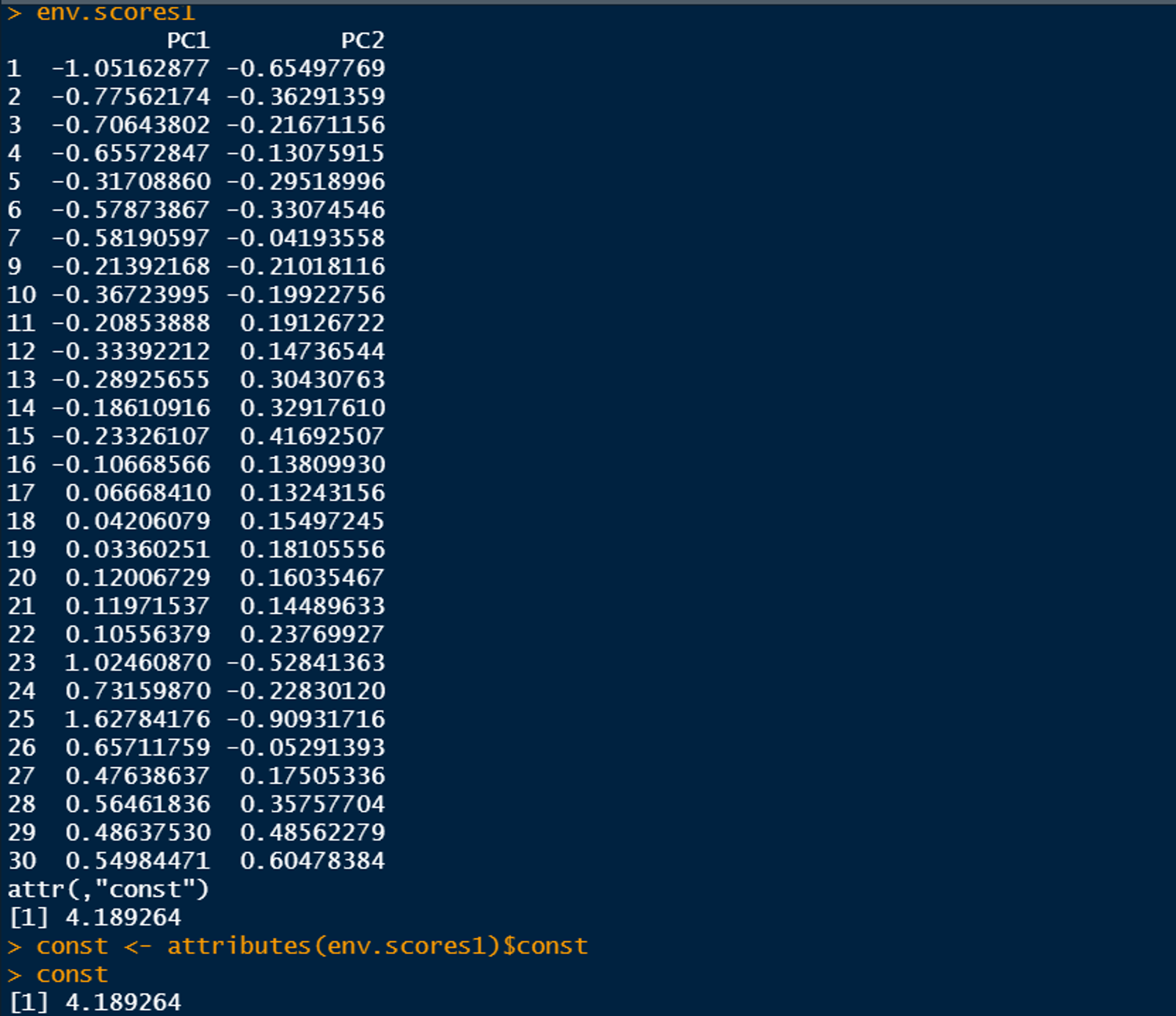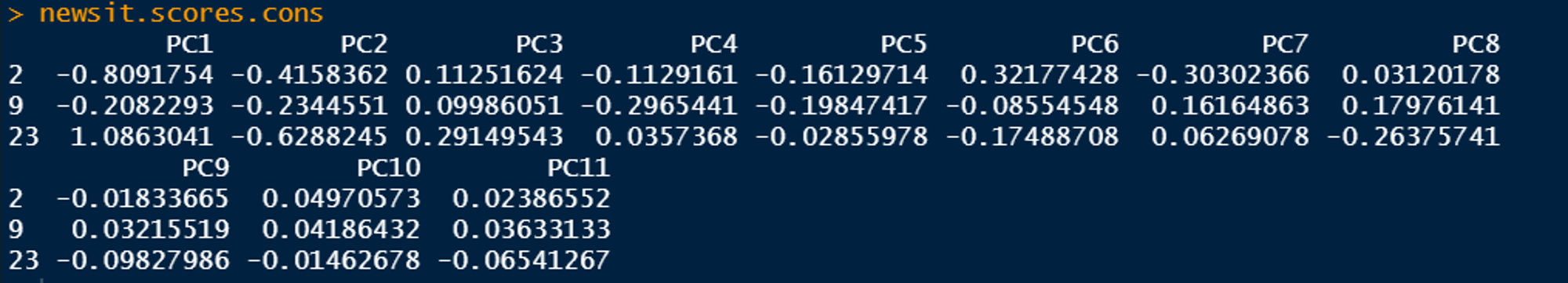墨滴fafu生信小蘑菇

2021/05/16  阅读：43  主题：自定义主题1

# 第五章 非约束排序2 主成分分析（PCA）

## 1. 主成分分析（PCA）概述

PCA排序分析的对象是离差矩阵，即包含方差和协方差的变量之间（相同量纲）的关联矩阵，或不同纲量的变量之间的相关系数矩阵。PCA致力于分析定量数据，展示欧氏距离和线性关系。因此，PCA通常不适合原始的物种多度数据分析。但是，对原始数据进行适当转化后的物种数据可以进行PCA。

## 2.使用rda()函数对Doubs环境数据进行PCA分析

Doubs数据集有11个定量环境变量。它们有什么相关性？从样方排序可以获得什么信息？ 由于环境变量具有不同的量纲，这里是计算基于相关矩阵的PCA。相关系数实际上也就是变量标准化后的协方差。

### 2.1 数据准备

setwd("E:/Rstudio_working/lai jian shan/DATA/Data")# 加载包，函数和数据library(ade4)library(vegan)library(gclus)library(ape)library(missMDA)library(FactoMineR)#加载本章后面部分所需要的函数source("cleanplot.pca.R")source("PCA.newr.R")source("CA.newr.R")# 导入Doubs 数据load("Doubs.RData")# 删除无物种数据的样方8spe <- spe[-8, ]env <- env[-8, ]spa <- spa[-8, ]# 导入 oribatid 甲螨数据load("mite.RData")

###2.2 基于相关矩阵的PCA

# 显示环境变量数据集的内容summary(env)
# 基于相关矩阵PCA# 参数scale=TRUE表示对变量进行标准化env.pca <- rda(env, scale = TRUE)env.pcasummary(env.pca) # 默认scaling 2#注意函数summary（）内的参数scaling，为绘制排序图所选择的标尺类型，与函数rda（）内数据标准化的参数scale无关。summary(env.pca, scaling = 1)#如果不想查看样方和物种坐标，可以将summary()里面设定参数axes=0summary(env.pca,axes = 0) 

PCA排序结果的解读:

1. 惯量（inertia）：在vegan包里，是数据“变差”的通用术语。在PCA里，“惯量”可以是变量总方差（基于协方差矩阵的PCA分析），也可以是相关矩阵的对角线数值和（基于相关矩阵的PCA）（例如本案例），即变量自相关系数的总和，也等于变量的数量（例如本案例中有11个变量，惯量等于11）。

2. 约束和非约束（constrained and unconstrained）：PCA是非约束排序（即不是被环境因子解释），所以这里显示“unconstrained”。

3. 特征根（eigenvalues）：是每个排序轴的重要性（方差）的指标，可以用特征根数值，也可以用占总变差的比例表示（每轴特征根除以总惯量）。

4. 标尺（（scaling）：请不要与rda（）函数内的变量标准化参数scale混淆*。“scaling”是指排序结果投影到排序空间的可视化方式*。一般的排序图需要同时展示对象和变量[称为“双序图”（biplot）]，但没有同时可视化对象和变量的最优化方法。一般有两种标尺模式，不同模式的排序图有不同解读，即关注点不同。下面给出每种模式最主要的特征。

• 1型标尺（scaling1）=距离双序图（distance biplot）特征向量被标准化为单位长度，关注的是对象之间的关系。①双序图中对象之间的距离近似于多维空间内的欧氏距离。②代表变量的箭头之间的夹角不反映变量之间的相关
• 2型标尺（scaling2）=相关双序图（correlation biplot）每个特征向量被标准化为特征根的平方根，关注的是变量之间的关系。①双序图中对象之间的距离不再近似于多维空间内的欧氏距离。②代表变量箭头之间的夹角反映变量之间的相关性
• 在这两种模式下，将对象点垂直投影到变量箭头上位置表示该变量在该对象内数值在所有样方内的排序位置。
• 选择建议：如果分析兴趣在于解读对象之间的关系，设定scaling=1。如果分析兴趣在于解读变量之间关系，设定scaling=2
• 折中标尺，scaling=3，也称为“对称标尺”，也是常被用到，包括通过样方和物种的特征根的平方根。这种不特别强调关注对象或是变量。这种折中标尺没有明确的解释规则。
5. Species scores：代表变量的箭头在排序图的坐标。由于历史的原因，在vegan包里，所有的响应变量都统称为物种，不管这些变量是否真的是物种。

6. Site scores对象在排序图的坐标。在vegan包里，所有的对象都统称为样方

### 2.3 提取、解读和绘制vegan包输出的PCA结果

vegan包输出的排序结果是一个复杂的实体，其元素的提取并不总是遵循R的基本规则。在R的控制台输入*？cca.object，可以打开解释rda（）和cca（）函数输出结果的帮助文件*。在帮助文件的最后案例（example）部分可以学到如何访问或提取排序结果某一部分。

2.3.1 特征根（Eigenvalues）

PCA不是统计检验，而是探索性分析方法，其目的是在低维空间尽可能多地展示数据主要趋势特征

1. **断棍模型:**主要原理是将单位长度的棍子随机分成与PCA轴数一样多的几段，然后将这些断棍按照长短依次赋予对应的轴（即最长的棍子赋予第一轴，第二长的赋予第二轴，依此类推）。

1. Kaiser-Guttman准则
?cca.object   #打开解释rda（）和cca（）函数输出结果的帮助文件*# 特征根(ev <- env.pca$CA$eig)# 碎石图和断棍模型dev.new(title = "PCA特征值的碎石图", noRStudioGD = TRUE)screeplot(env.pca, bstick = TRUE, npcs = length(env.pca$CA$eig))
# 断棍模型n <- length(ev)bsm <- data.frame(j=seq(1:n), p=0)bsm$p <- 1/nfor (i in 2:n) { bsm$p[i] = bsm$p[i-1] + (1/(n + 1 - i))}bsm$p <- 100*bsm$p/nbsm# 绘制每轴的特征根和方差百分比dev.new(width = 6, height = 12, title = "绘制每轴的特征根和方差百分比", noRStudioGD = TRUE)par(mfrow=c(2,1))barplot(ev, main="特征根", col="bisque", las=2abline(h=mean(ev), col="red") # 特征根平均值legend("topright", "平均特征根", lwd=1, col=2, bty="n")barplot(t(cbind(100*ev/sum(ev),bsm$p[n:1])), beside=TRUE,         main="% 变差", col=c("bisque",2), las=2)legend("topright", c("% 特征根", "断棍模型"),        pch=15, col=c("bisque",2), bty="n")

2.3.2样方和变量的双序图

# 使用biplot.rda()函数绘制排序图dev.new(width = 12,        height = 6,        title = "使用biplot.rda()函数生成的Doubs环境数据PCA分析双序图",         noRStudioGD = TRUE)par(mfrow = c(1, 2))biplot(env.pca, scaling = 1, main = "PCA - 1 型标尺")biplot(env.pca, main = "PCA - 2型标尺")  # 默认scaling =2
# 使用cleanplot.pca()函数绘图dev.new(width = 12,        height = 6,        title = " cleanplot.pca()函数生成的Doubs环境数据PCA分析双序图",         noRStudioGD = TRUE)par(mfrow = c(1, 2))cleanplot.pca(env.pca, scaling = 1, mar.percent = 0.08)cleanplot.pca(env.pca, scaling = 2, mar.percent = 0.04)

• 用biplot（），text（）和arrows（）等函数从PCA结果中提取我们感兴趣的样方或变量坐标，或者，使用作者编写cleanplot.pca（）函数直接让参数select.spe选择感兴趣的变量.
• vegan包现在接受了参数scaling=“sites”用于1型标尺，scaling=“species”用于2型标尺。vegan包涉及scaling的所有函数都可以用此规则。

1. 1型标尺双序图中的圆圈，称作平衡贡献圆。它的半径等于 d是双序图的轴数量（通常d=2）p是PCA的维度（即变量的个数）。平衡贡献圆的半径代表变量的向量长度对排序的平均贡献率。因此，在任何二维的排序图中，如果某个变量的箭头长度长于圆的半径，代表它对这个排序空间的贡献大于所有变量的平均贡献。在2型标尺不可能画出一个平衡贡献的圆圈，因为2型标尺是对“Mahalanobis”空间的投影，而不是欧氏空间。只有变量被标准化，且两两正交，才能准确获得变量的贡献，进而才能画出平衡贡献圆.
2. 1型标尺双序图（scaling 1 biplot）体现了从左到右的变化梯度，样方1-10可以归为第一组，从图中可以明显看出，第一组位于高海拔（ele）和陡坡（s1o）、低流量（dis）低硬度（har）的离源头距离（dfs）近的上游区域样方11-16可以归为第二组，处于高氧含量（oxy）和低硝酸盐浓度（nit）的区域第三组样方（17-22）比较密集，几乎处于所有变量中值的区域，也表明这些样方对前两轴的贡献不大。样方23-25所在区域也显示出磷酸盐浓度（pho）、铵浓度（amm）和生物需氧量（bod）具有最高值。总体来说，1型标尺双序图样方分组体现水质从营养贫瘠、氧丰富到富营养化，最后缺氧的变化。
3. 在2型标尺双序图（scaling 2 biplot）内，环境变量可以划分为不同的组。在图的左下角，显示海拔（ele）和坡度（slo）高度正相关，这两个变量也与离源头距离（dfs）、流量（dis）和钙浓度（har）组成的另外一组环境变量呈现明显负相关氧含量（oxy）与海拔（ele）和坡度（slo）呈正相关，但与磷酸盐浓度（nit）和铵浓度（amm）呈负相关，当然，也与生物需氧量（bod）负相关。双序图右半部显示的变量主要与河流的下游有关系，即流量（dis）和硬度（har）这组变量与离源头距离（dfs）这个变量和代表富营养化这组变量（即磷酸盐、铵浓度和生物需氧量）高度相关。硝酸盐浓度（nit）与这两组环境因子正相关。硝酸盐浓度（nit）与pH箭头几乎正交，显示它们的相关系数接近为0。pH的箭头很短，表明它对于前两轴的贡献并不大。但如果绘第一轴与第三轴的排序图可以发现pH对第三轴的贡献比较大。
4. 这个案例表明，双序图对于总结数据的主要特征是非常有用的。样方梯度分布和聚类、变量之间的相关性都比较清晰。基于相关矩阵的双序图（2型标尺）比直接查看变量之间的相关系数矩阵（可以通过输入cor（env）获得）更加直观。

#plot()函数生成的Doubs环境数据PCA分析双序图dev.new(width = 12,        height = 6,        title = " plot()函数生成的Doubs环境数据PCA分析双序图",         noRStudioGD = TRUE)par(mfrow = c(1, 2))plot(env.pca, scaling = 1)plot(env.pca, scaling = 2)                                                                                                                                                                             

# 使用ele, oxy, har, bod这4个变量dev.new(  title = "带有变量子集的PCA绘图 - cleanplot.pca",  width = 12,  height = 6,   noRStudioGD = TRUE)par(mfrow = c(1, 2))var.subset <- c(2, 6, 10, 11)cleanplot.pca(  env.pca,  scaling = 1,  select.spe = var.subset,  mar.percent = 0.10)cleanplot.pca(  env.pca,  scaling = 2,  select.spe = var.subset,  mar.percent = 0.04)
# 使用ele, oxy, har, bod这4个变量dev.new(  title = "带有变量子集的PCA绘图 - biplot vegan",  width = 14,  height = 7,  noRStudioGD = TRUE)par(mfrow = c(1, 2))# 标尺1var.sc1.sub <-  scores(env.pca,          scaling = 1,          display = "species")[c(2, 6, 10, 11), ]biplot(env.pca,       scaling = 1,       main = "PCA 标尺1",       type = "n")text(env.pca,   # 添加样方点     scaling = 1,     display = "sites",     cex = 0.7)arrows(  0,  0,  var.sc1.sub[, 1],  var.sc1.sub[, 2],  length = 0.10,  angle = 10,  col = "red")text(  var.sc1.sub[, 1],  var.sc1.sub[, 2],  labels = rownames(var.sc1.sub),  col = "red",  pos = 4)# 标尺2var.sc2.sub <- scores(env.pca,                       display = "species")[c(2, 6, 10, 11), ]biplot(env.pca, type = "n", main = "PCA 标尺2")text(env.pca,     scaling = 2,     display = "sites",     cex = 0.7)arrows(  0,  0,  var.sc2.sub[, 1],  var.sc2.sub[, 2],  length = 0.10,  angle = 10,  col = "red")text(  var.sc2.sub[, 1],  var.sc2.sub[, 2],  labels = rownames(var.sc2.sub),  col = "red",  pos = 4)

### 2.4 将新变量投影到PCA双序图中

# 去掉 oxy and bod 的PCAenv.pca2 <- rda(env[, -c(10, 11)], scale = TRUE)# 生成只有 oxy and bod 对的数据框（作为新变量）new.var <- env[, c(10, 11)]# 计算新变量的坐标（箭头尖端位置）new.vscores <-  predict(env.pca2,          type = "sp",          newdata = new.var,          scaling = 2)
# 计算新变量的坐标（箭头尖端位置）new.vscores <-  predict(env.pca2,          type = "sp",          newdata = new.var,          scaling = 2)# 绘制结果-2型标尺dev.new(  title = "主成分分析中的补充变量",  noRStudioGD = TRUE)biplot(env.pca2,       scaling = 2,       main = "主成分分析中的补充变量")arrows(  0,  0,  new.vscores[, 1],  new.vscores[, 2],  length = 0.05,  angle = 30,  col = "blue")text(  new.vscores[, 1],  new.vscores[, 2],  labels = rownames(new.vscores),  cex = 0.8,  col = "blue",  pos = 2)

• 将新变量投影到PCA结果中仅适用于新变量的数据预处理方式与已被用于PCA变量数据预处理方式要一致
• 在本案例中，原来PCA的变量进行标准化（scale=TRUE），但标准化是在PCA过程进行的，即提供给函数rda（）的数据是未标准化的变量。因为函数predict（）是从PCA输出中提取其信息（这里是env.pca2），这表示新的变量已经在“预测”过程中自动做了跟原始的PCA相同的数据标准化。
• 如果原始的PCA分析是在输入rda（）函数之前就做了数据转化，则新变量在给predict（）函数之前也得做相应的手动转化。如果是根据物种多度数据Hellinger转化后做PCA或任何涉及行的转化，新的物种数据中在输入PCA预测之前，都应该做相应的转化，即需要用相同的行的和以及平方根进行转化。

### 2.5 将新对象投影到PCA双序图中

stats程序包的另一个predict（）函数可以正确预测由其他函数做出的RDA的样方坐标。例如，我们使用stats 包里面的prcomp（）函数做缺失样方2、9和22的Doubs鱼类数据的PCA，然后再预测出缺失样方的坐标并投影到原来的排序图中。

#将新对象（样方）投影到PCA图#使用stats包里面的prcomp（）和predict（）做缺失样方2、9和22的PCA#并预测和投影缺失样方的坐标#去掉样方9和22的命令中行号标为8和22是因为我们已经去掉无物种的样方8#使用prcomp（）运算PCA#参数’scale.=TRUE'就是基于相关矩阵的PCA（变量标准化）#与rda（）函数中‘scale=TRUE’效果一样env.prcomp<-prcomp(env[-c(2,8,22),],scale.=TRUE)
dev.new(  title = "使用prcomp函数补充新对象",   noRStudioGD = TRUE)plot(  env.prcomp$x[ ,1], env.prcomp$x[ ,2],   type = "n",  main = "PCA 标尺1-补充新对象",  xlab = "PCA 1",  ylab = "PCA 2")abline(h = 0, col = "gray")abline(v = 0, col = "gray")text(  env.prcomp$x[ ,1], env.prcomp$x[ ,2],   labels = rownames(env[-c(2, 8, 22), ]))
# 计算新的样方坐标new.sit <- env[c(2, 8, 22), ]pca.newsit <- predict(env.prcomp, new.sit) # 将新样方投影到PCA图text(  pca.newsit[, 1],  pca.newsit[, 2],  labels = rownames(pca.newsit),  cex = 0.8,  col = "blue")

1. vegan包会对样方坐标统一乘以一个常数，所以必须通过手动的方法也对新样方坐标做同样的操作，这个常数可以通过scores（）获得。
2. 如果PCA运算的是标准化数后的数据，新样方数据中的变量也必须使用原始数据组的平均值和标准差进行标准化。

### 2.6 环境变量的PCA减去站点2、9和23

#基于使用vegan函数rda（）计算的PCAenv.small <- env[-c(2, 8, 22), ]env.pca3 <- rda(env.small, scale = TRUE)
# 用点2、8和22创建数据框new.sit <- env[c(2, 8, 22), ]# 计算新站点的得分# Extract Matrix U (在包vegan中叫做v)U.mat <- env.pca3$CA$v# 用PCA中使用的数据参数标准化新数据env.mean <- apply(env.small, 2, mean)env.sd <- apply(env.small, 2, sd)newsit.stand <- scale(new.sit, center = env.mean, scale = env.sd)
# 原始标尺1 新站点的分数newsit.scores <- newsit.stand %*% U.mat
#vegan评分的提取与检索# 标尺常数env.scores1 <-  scores(    env.pca,    display = "sites",    choices = c(1, 2),    scaling = 1  )const <- attributes(env.scores1)$const# 计算新站点的分数newsit.scores.cons <- newsit.scores / const图2.6-4图2.6-5 # 绘制结果-标尺1dev.new(title = "Supplementary sites in PCA", noRStudioGD = TRUE)biplot(env.pca3, scaling = 1)text( newsit.scores.cons[, 1], newsit.scores.cons[, 2], labels = rownames(newsit.scores.cons), cex = 0.8, col = "blue")# 手动计算 vegan 常数n <- nrow(env[-c(2, 8, 22), ])eigenv <- env.pca3$CA\$eigtot <- sum(eigenv)const <- ((n - 1) * tot) ^ 0.25

### 2.7 组合聚类分析结果和排序结果

1. 在排序图内用不同颜色去区分不同样方组
2. 将聚类树添加到排序图上jieg

#聚类组合分析结果和排序结果#使用环境变量数据对样方进行基于变量标准化后欧氏距离的Ward聚类分析env.w <- hclust(dist(scale(env)), "ward.D")# 裁剪聚类树，只保留4个聚类簇gr <- cutree(env.w, k = 4)grl <- levels(factor(gr))# 提取样方坐标，1型标尺sit.sc1 <- scores(env.pca, display = "wa", scaling = 1)
# 按照聚类分析的结果对样方进行标识和标色（1型标尺）dev.new(title = "排序和聚类", noRStudioGD = TRUE)p <- plot(  env.pca,  display = "wa",  scaling = 1,  type = "n",  main = "PCA （基于相关矩阵）+聚类簇")abline(v = 0, lty = "dotted")abline(h = 0, lty = "dotted")for (i in 1:length(grl)) {  points(sit.sc1[gr == i, ],         pch = (14 + i),         cex = 2,         col = i + 1)}text(sit.sc1, row.names(env), cex = 0.7, pos = 3)
#在排序图内增加聚类树ordicluster(p, env.w, col = "dark grey")# 人机互动图例legend(  locator(1),  paste("Cluster", c(1:length(grl))),  pch = 14 + c(1:length(grl)),  col = 1 + c(1:length(grl)),  pt.cex = 2)fafu生信小蘑菇

2021/05/16  阅读：43  主题：自定义主题1